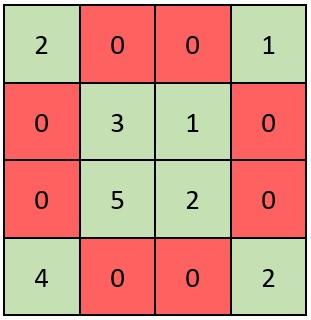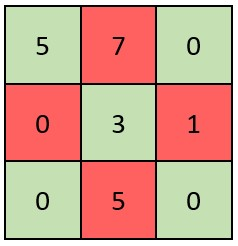2319. Check if Matrix Is X-Matrix

#### QUESTION:

A square matrix is said to be an X-Matrix if both of the following conditions hold:

All the elements in the diagonals of the matrix are non-zero. All other elements are 0. Given a 2D integer array grid of size n x n representing a square matrix, return true if grid is an X-Matrix. Otherwise, return false.

Example 1:``````Input: grid = [[2,0,0,1],[0,3,1,0],[0,5,2,0],[4,0,0,2]]
Output: true
Explanation: Refer to the diagram above.
An X-Matrix should have the green elements (diagonals) be non-zero and the red elements be 0.
Thus, grid is an X-Matrix.
``````

Example 2:``````Input: grid = [[5,7,0],[0,3,1],[0,5,0]]
Output: false
Explanation: Refer to the diagram above.
An X-Matrix should have the green elements (diagonals) be non-zero and the red elements be 0.
Thus, grid is not an X-Matrix.
``````

Constraints:

``````n == grid.length == grid[i].length
3 <= n <= 100
0 <= grid[i][j] <= 105
``````

#### SOLUTION:

``````class Solution {
func checkXMatrix(_ grid: [[Int]]) -> Bool {
var result:Bool = true
for indexI in grid.indices {
for indexJ in grid[indexI].indices {
if (indexI == indexJ || indexJ == grid.count - 1 - indexI) {
if (grid[indexI][indexJ] == 0) {
return false
}
} else {
if (grid[indexI][indexJ] != 0) {
return false
}
}
}
}
return result
}
}
``````